## Proc Transpose Non-Systematic Data

Hi,
I have a subtitle file, which I want to convert into data: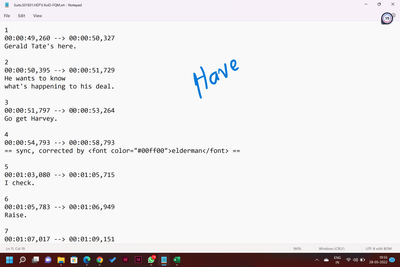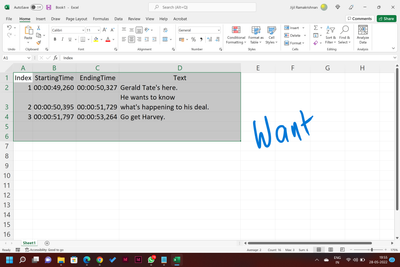As few entries have multiple lines, I seek some help.

Jijil Ramakrishnan

1 ACCEPTED SOLUTION

Accepted Solutions

## Re: Proc Transpose Non-Systematic Data

Seems pretty simple as the structure looks very regular (systematic actually).

Just keep reading comment lines until you hit an empty line.

``````data want;
infile have truncover end=eof;
length id 8 start end \$12 nrows 8 comment \$300 ;
input id / (start 2*end) (:) / ;
do nrows=1 by 1 until(_infile_=' ' or eof);
comment=catx('|',comment,_infile_);
input;
end;
run;``````

Result:

``````options nocenter ls=132 ps=60;

column id start end nrows comment;
define comment / width=80 flow;
run;``````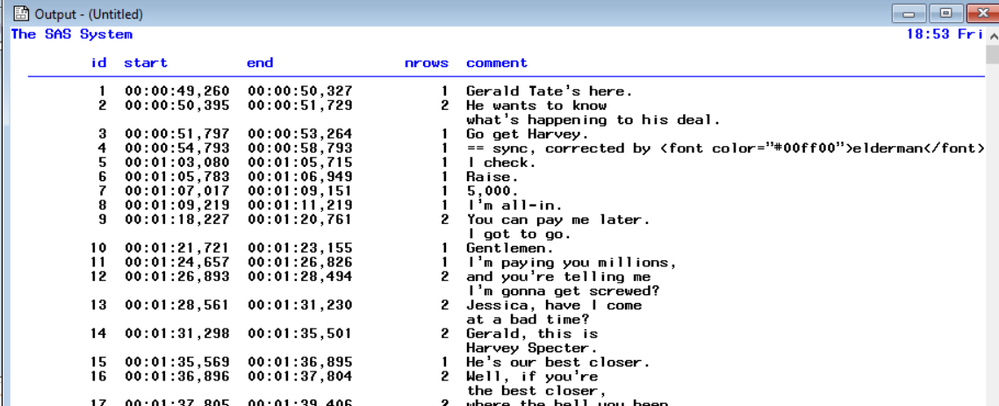10 REPLIES 10

## Re: Proc Transpose Non-Systematic Data

First a comment about your Want data set? You really do want to repeat the Index, and likely the start and end time values on each observation after reading the data. Otherwise any SORT is going to leave the text "orphaned" from the proper information about index for any of the multiple line bits.

The below example has had the , in the time values changed to . as I am not going to mess with my system settings to deal with foreign language conventions. The code should work with your data pointing to your text file with an infile statement. Or copy some of your example text and replace the datalines I used for testing.

```data example;
informat index 8. starttime endtime time15. text \$100.;
format starttime endtime time12.3;
retain index starttime endtime;
input @;
if input(_infile_,?? 8.) then do;
index =input(_infile_,8.);
input;
input starttime text endtime;
input ;
text=_infile_;
output;
end;
else if anyalpha(_infile_) then do;
text=_infile_;
output;
input;
end;
else input;

datalines;
1
00:00:49.260 --> 00:00:50.327
Gerald Tate's here.

2
00:00:50.395 --> 00:00:51.729
He wants to know
what's happening to his deal.

3
00:00:51.797 --> 00:00:53.264
Go get Harvey.

4
00:00:54.793 --> 00:00:58.793
== sync, corrected by <font color="#00ff00">elderman</font> ==
;

```

If you haven't used the trailing @ on input it holds the input buffer so it can be examined in the SAS automatic variable _infile_. So the code checks to see if a line is a valid number and if so assumes that is the index value, then reads the time values. Reuse of the TEXT variable to read the --> instead of dealing with fancier parsing of that line. Also assumes one line of text.

The check for the number value using the input function includes the ?? to suppress invalid data messages that would occur with the second text line in those sets such as on index=2.

## Re: Proc Transpose Non-Systematic Data

@ballardw
You are right, the Index must increment for every line of text.
How can I change the "2" into "3".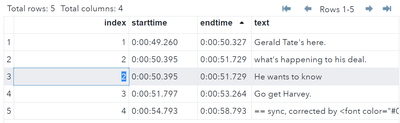Thank you!
Jijil

## Re: Proc Transpose Non-Systematic Data

I would say that you do NOT want change that "Index". Why? The second or other subsequent lines of text belong in the same "group".

This might be one of the times where combining different records so all that text is a single value make sense.

True!

## Re: Proc Transpose Non-Systematic Data

Seems pretty simple as the structure looks very regular (systematic actually).

Just keep reading comment lines until you hit an empty line.

``````data want;
infile have truncover end=eof;
length id 8 start end \$12 nrows 8 comment \$300 ;
input id / (start 2*end) (:) / ;
do nrows=1 by 1 until(_infile_=' ' or eof);
comment=catx('|',comment,_infile_);
input;
end;
run;``````

Result:

``````options nocenter ls=132 ps=60;

column id start end nrows comment;
define comment / width=80 flow;
run;``````## Re: Proc Transpose Non-Systematic Data

Thank you! This is so concise and efficient!Ksharp
Super User

## Re: Proc Transpose Non-Systematic Data

``````data have;
infile "c:\temp\subtitle.txt" encoding='utf-8' termstr=crlf length=len;
input temp \$varying200. len;
if missing(temp) then do;group+1;delete;end;
run;

data have2;
set have;
by group;
if first.group then n=0;
n+1;
if n>2 then n=3;
run;

data have3;
do until(last.n);
set have2;
by group n;
length want \$ 200;
want=catx(' ',want,temp);
end;
drop temp;
run;

proc transpose data=have3 out=have4 prefix=var;
by group;
id n;
var want;
run;

data want;
set have4(rename=(var1=index var3=text));
start_time=scan(var2,1,'-> ');
end_time=scan(var2,-1,'-> ');
drop group _name_ var2;
run;``````

## Re: Proc Transpose Non-Systematic Data

Thank you KShap!Ksharp
Super User

## Re: Proc Transpose Non-Systematic Data

Sorry. Tom,

I have to say Tom's code lost the last record.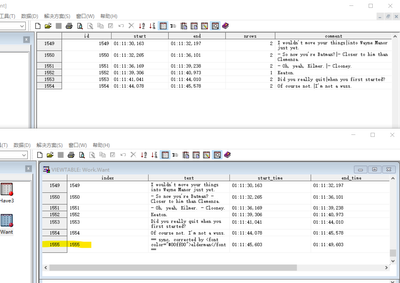## Re: Proc Transpose Non-Systematic Data

That just needs a trivial change avoid reading past the end of the file.

``````data want;
infile have truncover end=eof;
length id 8 start end \$12 nrows 8 comment \$300 ;
input id / (start 2*end) (:) ;
if eof then _infile_=' ';
else input ;
do nrows=1 by 1 while(_infile_ ne ' ');
comment=catx('|',comment,_infile_);
if eof then _infile_=' ';
else input;
end;
run;``````

Discussion stats
• 10 replies
• 549 views
• 1 like
• 4 in conversation